Thank you for visiting nature.com. You are using a browser version with limited support for CSS. To obtain the best experience, we recommend you use a more up to date browser (or turn off compatibility mode in Internet Explorer). In the meantime, to ensure continued support, we are displaying the site without styles and JavaScript.

# Towards fault-tolerant quantum computing with trapped ions

## Abstract

Today, ion traps are among the most promising physical systems for constructing a quantum device harnessing the computing power inherent in the laws of quantum physics1,2. For the implementation of arbitrary operations, a quantum computer requires a universal set of quantum logic gates. As in classical models of computation, quantum error correction techniques3,4 enable rectification of small imperfections in gate operations, thus enabling perfect computation in the presence of noise. For fault-tolerant computation5, it is believed that error thresholds ranging between 10−4 and 10−2 will be required—depending on the noise model and the computational overhead for realizing the quantum gates6,7,8—but so far all experimental implementations have fallen short of these requirements. Here, we report on a Mølmer–Sørensen-type gate operation9,10 entangling ions with a fidelity of 99.3(1)%. The gate is carried out on a pair of qubits encoded in two trapped calcium ions using an amplitude-modulated laser beam interacting with both ions at the same time. A robust gate operation, mapping separable states onto maximally entangled states is achieved by adiabatically switching the laser–ion coupling on and off. We analyse the performance of a single gate and concatenations of up to 21 gate operations.

## Main

For ion traps, all building blocks necessary for the construction of a universal quantum computer1 have been demonstrated over the past decade. Currently, the most important challenges consist of scaling up the present systems to a higher number of qubits and raising the fidelity of gate operations up to the point where quantum error correction techniques can be successfully applied. Although single-qubit gates are easily carried out with high quality, the realization of high-fidelity entangling two-qubit gates11,12,13,14,15,16 is much more demanding because the inter-ion distance is orders of magnitude bigger than the characteristic length scale of any state-dependent ion–ion interaction. Apart from quantum gates of the Cirac–Zoller type2,12, where a laser couples a single qubit with a vibrational mode of the ion string at a time, most other gate realizations entangling ions have relied on collective interactions of the qubits with the laser control fields11,13,14,15. These gate operations entangle transiently the collective pseudospin of the qubits with the vibrational mode and produce either a conditional phase shift17 or a collective spin flip9,10,18 of the qubits. Whereas the highest fidelity F=97% reported until now13 has been achieved with a conditional phase gate acting on a pair of hyperfine qubits in 9Be+, spin-flip gates have been limited so far to F≈85% (refs 11, 14). All of these experiments have used qubits encoded in hyperfine or Zeeman ground states and a Raman transition mediated by an electric-dipole transition for coupling the qubits. Whereas spontaneous scattering from the mediating short-lived levels degrades the gate fidelity owing to the limited amount of laser power available in current experiments19, this source of decoherence does not occur for optical qubits, that is, qubits encoded in a ground state and a metastable electronic state of an ion. In the experiment presented here, where the qubit comprises the states |S〉≡S1/2(m=1/2) and |D〉≡D5/2(m=3/2) of the isotope 40Ca+, spontaneous decay of the metastable state reduces the gate fidelity by less than 5×10−5.

A Mølmer–Sørensen gate inducing collective spin flips is achieved with a bichromatic laser field with frequencies ω±=ω0±δ, with ω0 being the qubit transition frequency and δ close to the vibrational mode frequency ν (Fig. 1). For optical qubits, the bichromatic field can be a pair of co-propagating lasers, which is equivalent to a single laser beam resonant with the qubit transition and amplitude-modulated with frequency δ. For a gate mediated by the axial centre-of-mass (COM) mode, the hamiltonian describing the laser–qubit interaction is given by $H = ℏ Ω e - i φ S + ( e - i ( δ t + ζ ) + e i ( δ t + ζ ) ) e i η ( a e - i v t + a † e i v t ) + h.c.$ Here, Sj=σj(1)+σj(2),j{+,−,x,y,z}, denotes a collective atomic operator constructed from Pauli spin operators σj(i) acting on ion i, and σ+(i)|Si=|Di. The operators aa annihilate and create phonons of the COM mode with Lamb–Dicke factor η. The optical phase of the laser field (with coupling strength Ω) is labelled φ, and the phase ζ accounts for a time difference between the start of the gate operation and the maximum of the amplitude modulation of the laser beam. In the Lamb–Dicke regime, and for φ=0, the gate operation is very well described by the propagator20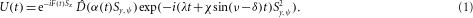Here, the operator to the right describes collective spin flips induced by the operator Sy,ψ=Sycosψ+Szsinψ, ψ=(4Ω/δ)cos ζ, and λη2Ω2/(νδ), χη2Ω2/(νδ)2. With α(t)=α0(ei(νδ)t−1), the displacement operator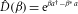accounting for the transient entanglement between the qubits and the harmonic oscillator becomes equal to the identity after the gate time τgate=2π/|νδ|. The operator eiF(t)Sx with F(t)=(2Ω/δ)(sin(δ t+φ)−sinφ) describes fast non-resonant excitations of the carrier transition that occur in the limit of short gates when Ωδ no longer strictly holds. Non-resonant excitations are suppressed by intensity-shaping the laser pulse so that the Rabi frequency Ω(t) is switched on and off smoothly. Moreover, adiabatic switching makes the collective spin-flip operator independent of ζ as Sy,ψSy for Ω→0. To achieve adiabatic following, it turns out to be sufficient to switch on the laser within 2.5 oscillation periods of the ions’ axial COM mode. When the laser is switched on adiabatically, equation (1) can be simplified by dropping the factor eiF(t)Sx and replacing Sy,ψ by Sy. To realize an entangling gate of duration τgate described by the unitary operator Ugate=exp(−i(π/8)Sy2), the laser intensity needs to be set such that η Ω≈|δν|/4.

Two 40Ca+ ions are confined in a linear trap21 with axial and radial COM mode frequencies of νaxial/2π=1.23 MHz and νradial/2π=4 MHz, respectively. After Doppler cooling and frequency-resolved optical pumping22 in a magnetic field of 4 G, the two axial modes are cooled close to the motional ground state (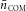,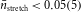). Both ions are now initialized to |S S〉 with a probability of more than 99.8%. Then, the gate operation is carried out, followed by an optional carrier pulse for analysis. Finally, we measure the probability pk of finding k ions in the |S〉 state by detecting light scattered on the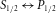dipole transition with a photomultiplier for 3 ms. The error in state detection due to spontaneous decay from the D state is estimated to be less than 0.15%. Each experimental cycle is synchronized with the frequency of the a.c.-power line and repeated 50–200 times. The laser beam carrying out the entangling operation is controlled by a double-pass acousto-optic modulator, which enables setting the frequency ωL and phase φ of the beam. By means of a variable gain amplifier, we control the radiofrequency input power and hence the intensity profile of each laser pulse. To generate a bichromatic light field, the beam is passed through another acousto-optic modulator in single-pass configuration that is driven simultaneously by two radiofrequency signals with difference frequency δ/π (see the first paragraph of the Methods section). Phase coherence of the laser frequencies is maintained by phase-locking all radiofrequency sources to an ultrastable quartz oscillator. We use 1.8 mW average light power focused down to a spot size of 14 μm gaussian beam waist illuminating both ions from an angle of 45 with equal intensity to achieve the Rabi frequencies Ω/(2π)≈110 kHz required for carrying out a gate operation with (νδ)/(2π)=20 kHz and η=0.044. To make the bichromatic laser pulses independent of the phase ζ, the pulse is switched on and off by using Blackman-shaped pulse slopes of duration τr=2 μs.

Multiple application of the bichromatic pulse of duration τgate ideally maps the state |S S〉 to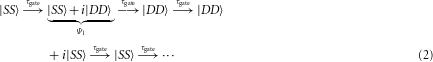up to global phases. Maximally entangled states occur at instances τm=m·τgate (m=1,3,…). A similar mapping of product states onto Bell states and vice versa also occurs when starting from state |S D〉. To assess the fidelity of the gate operation, we adapt the strategy first applied in refs 11, 13 consisting of measuring the fidelity of Bell states created by a single application of the gate to the state |S S〉 (Fig. 2a). The fidelity F=〈Ψ1|ρexp|Ψ1〉=(ρS S,S Sexp+ρD D,D Dexp)/2+ImρD D,S Sexp, with the density matrix ρexp describing the experimentally produced qubits’ state, is inferred from measurements on a set of 42,400 Bell states continuously produced within a measurement time of 35 min. Fluorescence measurements on 13,000 Bell states reveal that ρS S,S Sexp+ρD D,D Dexp=p2+p0=0.9965(4). The off-diagonal element ρD D,S Sexp is determined by measuring P(φ)=〈σφ(1)σφ(2)〉 for different values of φ, where σφ=σxcosφ+σysinφ, by applying (π/2)φ pulses to the remaining 29,400 states and measuring p0+p2p1 to obtain the parity 〈σz(1)σz(2)〉. The resulting parity oscillation P(φ) shown in Fig. 2b is fitted with a function Pfit(φ)=Asin(2φ+φ0) that yields A=2|ρD D,S Sexp|=0.990(1). Combining the two measurements, we obtain the fidelity F=99.3(1)% for the Bell state Ψ1.

A wealth of further information is obtained by studying the state dynamics under the action of the gate hamiltonian (see equation (2)). Starting from state |S S〉, Fig. 3 shows the time evolution of the state populations for pulse lengths equivalent to up to 17 gate times. The ions are entangled and disentangled consecutively up to nine times, the populations closely following the predicted unitary evolution of the propagator (1) for ζ=0 shown in Fig. 3 as solid lines.

To study sources of gate imperfections we measured the fidelity of Bell states obtained after a pulse length τm for up to m=21 gate operations. The sum of the populations p0(t)+p2(t) does not return perfectly to one at times τm as shown in Fig. 4 but decreases by about 0.0022(1) per gate. This linear decrease could be explained by resonant spin-flip processes caused by spectral components of the qubit laser that are far outside the laser’s linewidth of 20 Hz (ref. 21) (see the Methods section). The figure also shows the amplitude of parity fringe pattern scans at odd integer multiples of τgate similar to the one in Fig. 2b. The gaussian shape of the amplitude decay is consistent with variations in the coupling strength Ω that occur from one experiment to the next (see the Methods section).

The observed Bell-state infidelity of 7×10−3 indicates that the gate operation has an infidelity below the error threshold required by some models of fault-tolerant quantum computation6,7,8 (an indication to be confirmed by full quantum gate tomography16 in future experiments). However, further experimental advances will be needed before fault-tolerant computation will become a reality as the overhead implied by these models is considerable. Nevertheless, in addition to making the implementation of quantum algorithms with tens of entangling operations look realistic, the gate presented here also opens interesting perspectives for generating multiparticle entanglement23 by a single laser interacting with more than two qubits at once. For the generation of N-qubit Greenberger–Horne–Zeilinger states, there exist no constraints on the positioning of ions in the bichromatic beam that otherwise made generation of Greenberger–Horne–Zeilinger states beyond N=6 difficult in the experiment with hyperfine qubits described in ref. 24. Although the bichromatic force lacks a strong spatial modulation that would enable tailoring of the gate interaction by choosing particular ion spacings25,26, more complex multiqubit interactions could be engineered by interleaving entangling laser pulses addressing all qubits with a focused laser inducing phase shifts in single qubits. Akin to nuclear magnetic resonance techniques, this method should enable refocusing of unwanted qubit–qubit interactions27 and open the door to a wide variety of entangling multiqubit interactions.

## Methods

### a.c.-Stark-shift compensation

The red- and the blue-detuned frequency components ω± of the bichromatic light field cause dynamic (a.c.-) Stark shifts by non-resonant excitation on the carrier and the first-order sidebands that exactly cancel each other if the corresponding laser intensities I± are equal. The remaining a.c.-Stark shift due to other Zeeman transitions and far-detuned dipole transitions amounts to 7 kHz for a gate time τgate=50 μs. These shifts could be compensated by using an extra far-detuned light field28 or by properly setting the intensity ratio I+/I. We use the latter technique, which makes the coupling strengths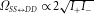,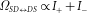slightly unequal. However, the error is insignificant asin our experiments.

### Sources of gate infidelity

A bichromatic force with time-dependent Ω(t) acting on ions prepared in an eigenstate of Sy creates coherent states α(t) following trajectories in phase space that generally do not close20,29. For the short rise times used in our experiments, this effect can be made negligibly (<10−4) small by slightly increasing the gate time.

Spin flips induced by incoherent off-resonant light of the bichromatic laser field reduce the gate fidelity. A beat frequency measurement between the gate laser and a similar independent laser system that was spectrally filtered indicates that a fraction γ of about 2×10−7 of the total laser power is contained in a 20 kHz bandwidth B around the carrier transition when the laser is tuned close to a motional sideband. A simple model predicts spin flips to cause a gate error with probability pflip=(πγ|νδ|)/(2η2B). This would correspond to a probability pflip=8×10−4, whereas the measured state populations shown in Fig. 4 would be consistent with pflip=2×10−3. Spin-flip errors could be further reduced by two orders of magnitude by spectrally filtering the laser light and increasing the trap frequency ν/(2π) to above 2 MHz where noise caused by the laser frequency stabilization is much reduced.

Imperfections due to low-frequency noise randomly shifting the laser frequency ωL with respect to the atomic transition frequency ω0 were estimated from Ramsey measurements on a single ion showing that an average frequency deviation σ(ωL−ω0)/(2π)=160 Hz occurred. From numerical simulations, we infer that for a single gate operation this frequency uncertainty gives rise to a fidelity loss of 0.25% (an infidelity of 10−4 would require σ(ωL−ω0)/(2π)=30 Hz). In our parity oscillation experiments shown in Figs 2b and 4, however, this loss is not directly observable because a small error in the frequency of the bichromatic laser beam carrying out the gate operation is correlated with a similar frequency error of the carrier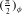pulse probing the entanglement produced by the gate so that the phase φ of the analysing pulse with respect to the qubit state remains well defined.

Variations in the coupling strength δ Ω induced by low-frequency laser intensity noise and thermally occupied radial modes were inferred from an independent measurement by recording the amplitude decay of carrier oscillations. Assuming a gaussian decay, we find a relative variation of δ Ω/Ω=1.4(1)×10−2. For m entangling gate operations, the loss of fidelity is approximately given by 1−F=(πm/2)2(δ Ω/Ω)2 and contributes with 5×10−4 to the error of a single gate operation. For the multiple gate operations shown in Fig. 4, this source of noise explains the gaussian decay of the parity fringe amplitude, whereas laser frequency noise reduces the fringe amplitude by less than 1% even for 21 gate operations. In combination with error estimates for state preparation, detection and laser noise, the analysis of multiple gates provides us with a good understanding of the most important sources of gate infidelity.

## References

1. 1

Nielsen, M. A. & Chuang, I. L. Quantum Computation and Quantum Information (Cambridge Univ. Press, Cambridge, 2000).

2. 2

Cirac, J. I. & Zoller, P. Quantum computations with cold trapped ions. Phys. Rev. Lett. 74, 4091–4094 (1995).

3. 3

Shor, P. W. Scheme for reducing decoherence in quantum computer memory. Phys. Rev. A 52, R2493–R2496 (1995).

4. 4

Steane, A. M. Error correcting codes in quantum theory. Phys. Rev. Lett. 77, 793–797 (1996).

5. 5

Shor, P. W. 37th Symposium on Foundations of Computing 56–65 (IEEE Computer Society Press, Washington DC, 1996).

6. 6

Knill, E. Quantum computing with realistically noisy devices. Nature 434, 39–44 (2005).

7. 7

Raussendorf, R. & Harrington, J. Fault-tolerant quantum computation with high threshold in two dimensions. Phys. Rev. Lett. 98, 190504 (2007).

8. 8

Reichardt, B. W. Improved ancilla preparation scheme increases fault-tolerant threshold. Preprint at &lt;http://arxiv.org/abs/quant-ph/0406025v1&gt; (2004).

9. 9

Sørensen, A. & Mølmer, K. Quantum computation with ions in thermal motion. Phys. Rev. Lett. 82, 1971–1974 (1999).

10. 10

Sørensen, A. & Mølmer, K. Entanglement and quantum computation with ions in thermal motion. Phys. Rev. A 62, 022311 (2000).

11. 11

Sackett, C. A. et al. Experimental entanglement of four particles. Nature 404, 256–259 (2000).

12. 12

Schmidt-Kaler, F. et al. Realization of the Cirac–Zoller controlled-NOT quantum gate. Nature 422, 408–411 (2003).

13. 13

Leibfried, D. et al. Experimental demonstration of a robust, high-fidelity geometric two ion-qubit phase gate. Nature 422, 412–415 (2003).

14. 14

Haljan, P. C. et al. Entanglement of trapped-ion clock states. Phys. Rev. A 72, 062316 (2005).

15. 15

Home, J. P. et al. Deterministic entanglement and tomography of ion spin qubits. New J. Phys. 8, 188 (2006).

16. 16

Riebe, M. et al. Process tomography of ion trap quantum gates. Phys. Rev. Lett. 97, 220407 (2006).

17. 17

Milburn, G. J., Schneider, S. & James, D. F. V. Ion trap quantum computing with warm ions. Fortschr. Phys. 48, 801–810 (2000).

18. 18

Solano, E., de Matos Filho, R. L. & Zagury, N. Deterministic Bell states and measurement of the motional state of two trapped ions. Phys. Rev. A 59, R2539–R2543 (1999).

19. 19

Ozeri, R. et al. Errors in trapped-ion quantum gates due to spontaneous photon scattering. Phys. Rev. A 75, 042329 (2007).

20. 20

Roos, C. F. Ion trap quantum gates with amplitude-modulated laser beams. New J. Phys. 10, 013002 (2008).

21. 21

Benhelm, J. et al. Measurement of the hyperfine structure of the S1/2–D5/2 transition in 43Ca+. Phys. Rev. A 75, 032506 (2007).

22. 22

Roos, C. F., Chwalla, M., Kim, K., Riebe, M. & Blatt, R. ‘Designer atoms’ for quantum metrology. Nature 443, 316–319 (2006).

23. 23

Mølmer, K. & Sørensen, A. Multiparticle entanglement of hot trapped ions. Phys. Rev. Lett. 82, 1835–1838 (1999).

24. 24

Leibfried, D. et al. Creation of a six-atom ‘Schrödinger cat’ state. Nature 438, 639–642 (2005).

25. 25

Chiaverini, J. et al. Realization of quantum error correction. Nature 432, 602–605 (2004).

26. 26

Reichle, R. et al. Experimental purification of two-atom entanglement. Nature 443, 838–841 (2006).

27. 27

Vandersypen, L. M. K. & Chuang, I. L. NMR techniques for quantum control and computation. Rev. Mod. Phys. 76, 1037–1069 (2004).

28. 28

Häffner, H. et al. Precision measurement and compensation of optical Stark shifts for an ion-trap quantum processor. Phys. Rev. Lett. 90, 143602 (2003).

29. 29

Leibfried, D., Knill, E., Ospelkaus, C. & Wineland, D. J. Transport quantum logic gates for trapped ions. Phys. Rev. A 76, 032324 (2007).

## Acknowledgements

We gratefully acknowledge the support of the European network SCALA and the Disruptive Technology Office and the Institut für Quanteninformation GmbH. We thank R. Gerritsma and F. Zähringer for help with the experiments.

## Author information

Authors

### Corresponding author

Correspondence to Christian F. Roos.

## Rights and permissions

Reprints and Permissions

Benhelm, J., Kirchmair, G., Roos, C. et al. Towards fault-tolerant quantum computing with trapped ions. Nature Phys 4, 463–466 (2008). https://doi.org/10.1038/nphys961

• Accepted:

• Published:

• Issue Date:

• ### Highlighting photonics: looking into the next decade

• Zhigang Chen
• Mordechai Segev

eLight (2021)

• ### A random-walk benchmark for single-electron circuits

• David Reifert
• Martins Kokainis
• Niels Ubbelohde

Nature Communications (2021)

• ### Quantum interference device for controlled two-qubit operations

• Niels Jakob Søe Loft
• Morten Kjaergaard
• Nikolaj T. Zinner

npj Quantum Information (2020)

• ### NOON state generation beyond the Lamb–Dicke limit in trapped-ion systems

• Xin-Jie Huang
• Lei Chen
• Zhi-Rong Zhong

Quantum Information Processing (2020)

• ### Realisation of high-fidelity nonadiabatic CZ gates with superconducting qubits

• Shaowei Li
• Anthony D. Castellano
• Jian-Wei Pan

npj Quantum Information (2019)

## Search